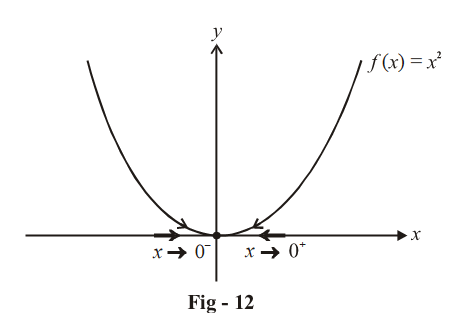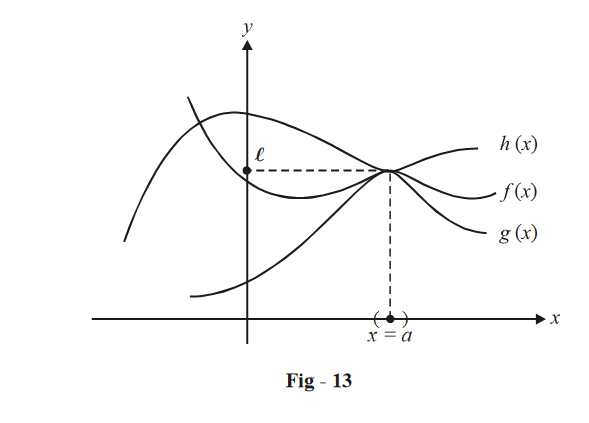# Introduction To Evaluating Limits

Go back to  'LCD'

Now that we’ve had an intuitive introduction to limits, how do we go about evaluating limits for arbitrary functions?

For example, consider \begin{align}f\left( x \right) = \frac{{\sin x}}{x}\end{align}. How does this function behave as \begin{align}x \to 0\end{align}? (This, as we have seen in the previous section, is an indeterminate form).

Before moving any further, one should note that if a function f(x) is determinate at x = a and ‘continuous’at x = a, implying that the graph of f(x) has no ‘break’ at ‘x = a’ (and in it’s neighbourhood), the limit of f(x) at x = a can be evaluated by direct substitution. That is,

$\mathop {\lim }\limits_{x \to a} f\left( x \right) = f\left( a \right)$

This will become obvious once we consider an example. Take  $$f\left( x \right) = {\rm{ }}{x^2}$$Consider $$\mathop {\lim }\limits_{x \to 0} f\left( x \right) = \mathop {\lim }\limits_{x \to 0} {x^2}$$. From the graph, we see that since $$f\left( x \right) = {\rm{ }}{x^2}$$ is ‘continuous’ at x = 0 (it has no break), f(x) will approach the same value from both sides, equal to f(0)

Hence,

$\mathop {\lim }\limits_{x \to 0} {x^2} = \mathop {\lim }\limits_{x \to {0^ - }} {x^2} = {\text{ LHL}} = \mathop {\lim }\limits_{x \to {0^ + }} {x^2} = {\text{RHL}} = f\left( 0 \right) = 0$

Thus, any determinate limit can be evaluated by direct substitution of x = a. (The function needs to have no breaks at x = a)

For example:

$$\mathop {\lim }\limits_{x \to 1} \left( {{x^2} + 2} \right) = 1 + 2 = 3$$

$$\mathop {\lim }\limits_{x \to 0} \left( {{x^3} + 4x + 5} \right) = 0 + 0 + 5 = 5$$

$$\mathop {\lim }\limits_{x \to \pi } \,\,\sin \,x = \sin \,\pi = 0$$

What about $$\mathop {\lim }\limits_{x \to 1} \left[ x \right]?$$

$$LHL = {\rm{ }}\mathop {\lim }\limits_{x \to {1^ - }} \left[ x \right] = 0$$

$$RHL = {\rm{ }}\mathop {\lim }\limits_{x \to {1^ + }} \left[ x \right] = 1$$

$$LHL{\rm{ }} \ne {\rm{ }}RHL$$

The limit does not exist ( f(x) is discontinuous at the point x = 1)

Note: Do not confuse continuity with existence of limits. Existence of limit at x = a implies LHL = RHL Continuity of f(x) at x = a implies LHL = RHL = f(a). We will soon study continuity in more detail.

The issue now is how to evaluate limits for indeterminate forms. This is the subject of the next section (where some standard indeterminate limits will be evaluated).

For now, observe some general rules pertaining to limits. These are more or less self explanatory.

Let $$\mathop {\lim }\limits_{x \to a} f\left( x \right) = l$$ and $$\mathop {\lim }\limits_{x \to a} g\left( x \right) = m.$$

If l, m exist, then:

(i) $$\mathop {\lim }\limits_{x \to a} \left\{ {f\left( x \right) \pm g\left( x \right)} \right\} = \left\{ {\mathop {\lim }\limits_{x \to a} f\left( x \right)} \right\} \pm \left\{ {\mathop {\lim }\limits_{x \to a} g\left( x \right)} \right\} = l \pm m$$

(ii) $$\mathop {\lim }\limits_{x \to a} \left\{ {f\left( x \right) \cdot g\left( x \right)} \right\} = \left\{ {\mathop {\lim }\limits_{x \to a} f\left( x \right)} \right\} \cdot \left\{ {\mathop {\lim }\limits_{x \to a} g\left( x \right)} \right\} = l \cdot m$$

(iii) \begin{align}\mathop {\lim }\limits_{x \to a} \left\{ {\frac{{f\left( x \right)}}{{g\left( x \right)}}} \right\} = \frac{{\left\{ {\mathop {\lim }\limits_{x \to a} f\left( x \right)} \right\}}}{{\left\{ {\mathop {\lim }\limits_{x \to a} g\left( x \right)} \right\}}} = \frac{l}{m}\;\;{\rm{if}}\,\,\,m \ne 0\end{align}

(iv) $$\mathop {\lim }\limits_{x \to a} \left\{ {K\,f\left( x \right)} \right\} = K\left\{ {\mathop {\lim }\limits_{x \to a} f\left( x \right)} \right\} = Kl$$

(v) $$\mathop {\lim }\limits_{x \to a} \left| {f\left( x \right)} \right| = \left| {\mathop {\lim }\limits_{x \to a} f\left( x \right)} \right|\,\, = \,\,\left| l \right|$$

(vi) $$\mathop {\lim }\limits_{x \to a} {\left( {f\left( x \right)} \right)^{g\left( x \right)}} = {\left( {\mathop {\lim }\limits_{x \to a} f\left( x \right)} \right)^{\left( {\mathop {\lim }\limits_{x \to a} g\left( x \right)} \right)}} = {l^m},$$ if the latter is defined.

(vii) $$\mathop {\lim }\limits_{x \to a} f\left( {g\left( x \right)} \right) = f\left( {\mathop {\lim }\limits_{x \to a} g\left( x \right)} \right) = f\left( m \right)$$

For example,

$$\mathop {\lim }\limits_{x \to a} \left( {\log f\left( x \right)} \right) = \log \left( {\mathop {\lim }\limits_{x \to a} f\left( x \right)} \right)$$

$$\mathop {\lim }\limits_{x \to a} \,\,{e^{f\left( x \right)}} = {e^{\mathop {\lim }\limits_{x \to a} f\left( x \right)}}$$

There needs to be a slight addition to this rule. Can you see what?

Consider $$f\left( x \right) = [x]\;and\;g{\rm{ }}\left( x \right){\rm{ }} = {\rm{ }}{x^3}$$

Then $$\mathop {\lim }\limits_{x \to 0} f\left( {g\left( x \right)} \right) = \mathop {\lim }\limits_{x \to 0} \left[ {{x^3}} \right].$$

Is this equal to $$\left[ {\mathop {\lim }\limits_{x \to 0} \,{x^3}} \right]?$$

Does the former limit even exist?

Based on the answers to these questions, modify the rule above.

(viii) We now come to an interesting rule, called the ‘Sandwich Rule’. As the name suggests, this rule describes the behaviour of a function f(x) ‘sandwiched’ between two different functions g(x) and h(x). At x = a, if g(x) and h(x), tend to the same value l, and f(x) is sandwiched between g(x) and h(x), it will obviously also tend to the same value l.For the graph above, in the neighbourghood of x = a, we see that the following always holds:

$$g\left( x \right) \le f\left( x \right) \le h\left( x \right)$$

or f(x) is sandwiched between g(x) and h(x).

Also, since $$\mathop {\lim }\limits_{x \to a} g\left( x \right) = \mathop {\lim }\limits_{x \to a} h\left( x \right) = l,$$ we have $$\mathop {\lim }\limits_{x \to a} f\left( x \right) = l$$

This is the sandwich theorem.

Let us now move on to the evaluation of some standard indeterminate limits. A good grasp on these limits will help you evaluate almost all limits with ease.